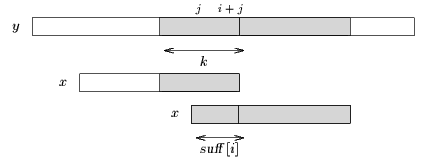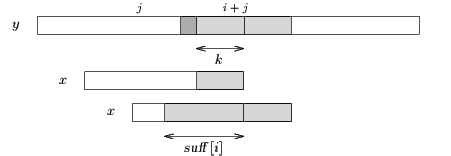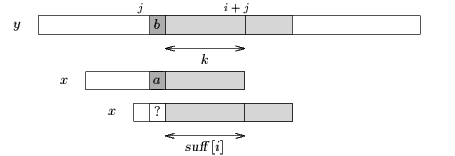Name
• String
• Substring
• Search
Edit

The Boyer-Moore algorithm is difficult to analyze because after each attempt it forgets all the characters it has already matched. Apostolico and Giancarlo designed an algorithm which remembers the length of the longest suffix of the pattern ending at the right position of the window at the end of each attempt. These information are stored in a table skip.

Let us assume that during an attempt at a position less than j the algorithm has matched a suffix of x of length k at position i+j with 0 < i < m then skip[i+j] is equal to k. Let suff[i], fori < m be equal to the length of the longest suffix of x ending at the position i in x (see chapter Boyer-Moore algorithm).

During the attempt at position j, if the algorithm compares successfully the factor of the text y[i+j+1 .. j+m-1]

then four cases arise:Case 1: k > suff[i] and suff[i]=i+1. It means that an occurrence of x is found at position j and skip[j+m-1] is set to m (see figure 15.1). A shift of length per(x) is performed.Figure 15.1, an occurrence of x is found.Case 2: k > suff[i] and suff[i]i. It means that a mismatch occurs between characters x[i-suff[i]] and y[i+j-suff[i]] and skip[j+m-1] is set to m-1-i+suff[i] (see figure 15.2). A shift is performed using bmBc[y[i+j-suff[i]]] and bmGs[i-suff[i]+1].Figure 15.2, a mismatch occurs between y[i+j-suff[i]] and x[i-suff[i]].Case 3: k < suff[i]. It means that a mismatch occurs between characters x[i-k] and y[i+j-k] and skip[j+m-1] is set to m-1-i+k (see figure 15.3). A shift is performed using bmBc[y[i+j-k]]and bmGs[i-k+1].Figure 15.3, a mismatch occurs between y[i+j-k] and x[i-k].Case 4: k=suff[i]. This is the only case where a "jump" has to be done over the text factor y[i+j-k+1 .. i+j] in order to resume the comparisons between the characters y[i+j-k] and x[i-k] (see figure 15.4).Figure 15.4, ab.

In each case the only information which is needed is the length of the longest suffix of x ending at position i on x.

The Apostolico-Giancarlo algorithm use two data structures:a table skip which is updated at the end of each attempt j in the following way: skip[j+m-1]=max{ k : x[m-k .. m-1]=y[j+m-k .. j+m-1]}the table suff used during the computation of the table bmGs: for 1i < msuff[i]=max{k : x[i-k+1 .. i]=x[m-k .. m-1]}

The complexity in space and time of the preprocessing phase of the Apostolico-Giancarlo algorithm is the same than for the Boyer-Moore algorithm: O(m+).

During the search phase only the last m informations of the table skip are needed at each attempt so the size of the table skip can be reduced to O(m).

The Apostolico-Giancarlo algorithm performs in the worst case at mostn text character comparisons.

Main features:

• variant of the Boyer-Moore algorithm;
• preprocessing phase in O(m+) time and space complexity;
• searching phase in O(n) time complexity;
• n comparisons in the worst case;

C